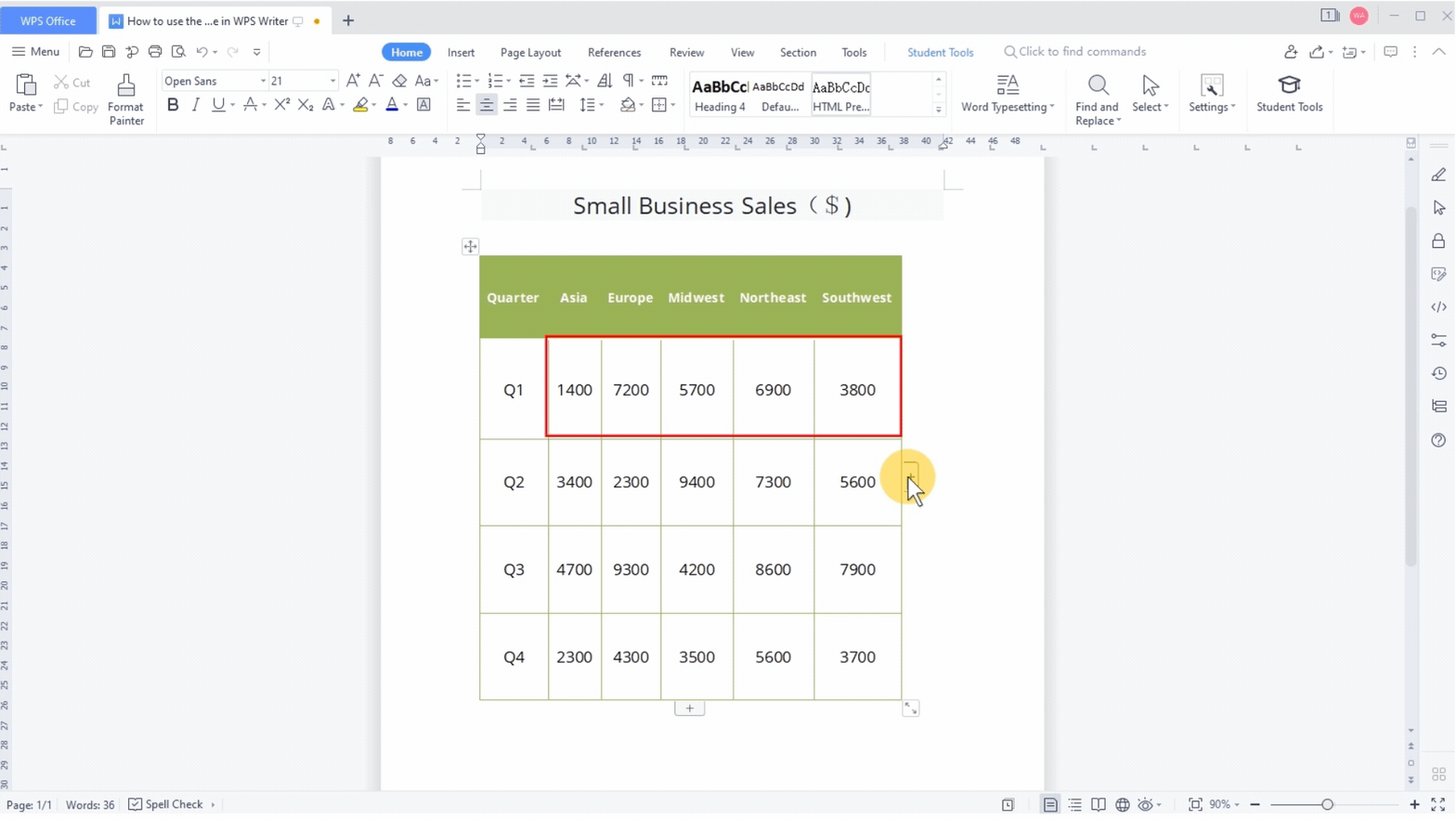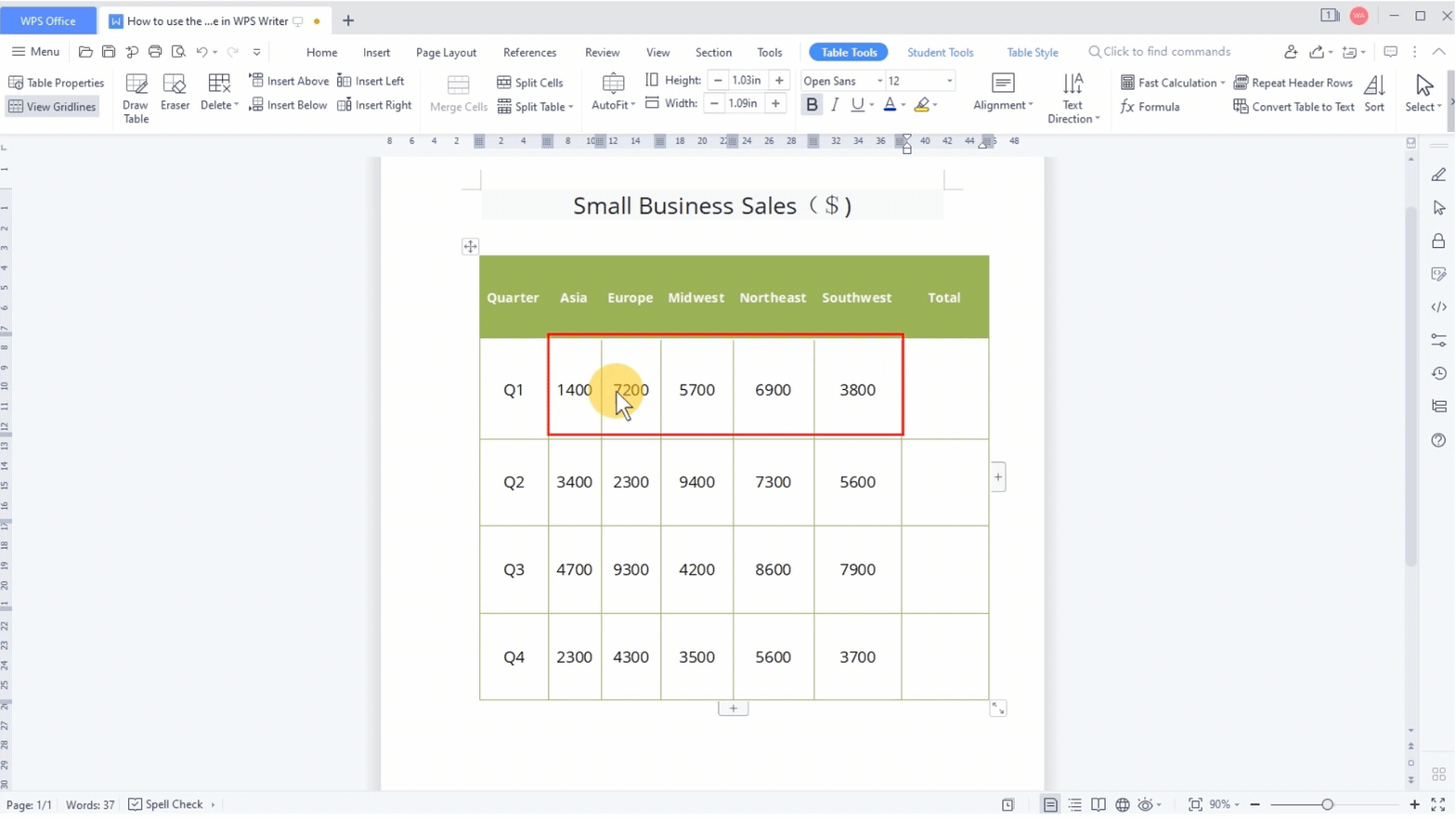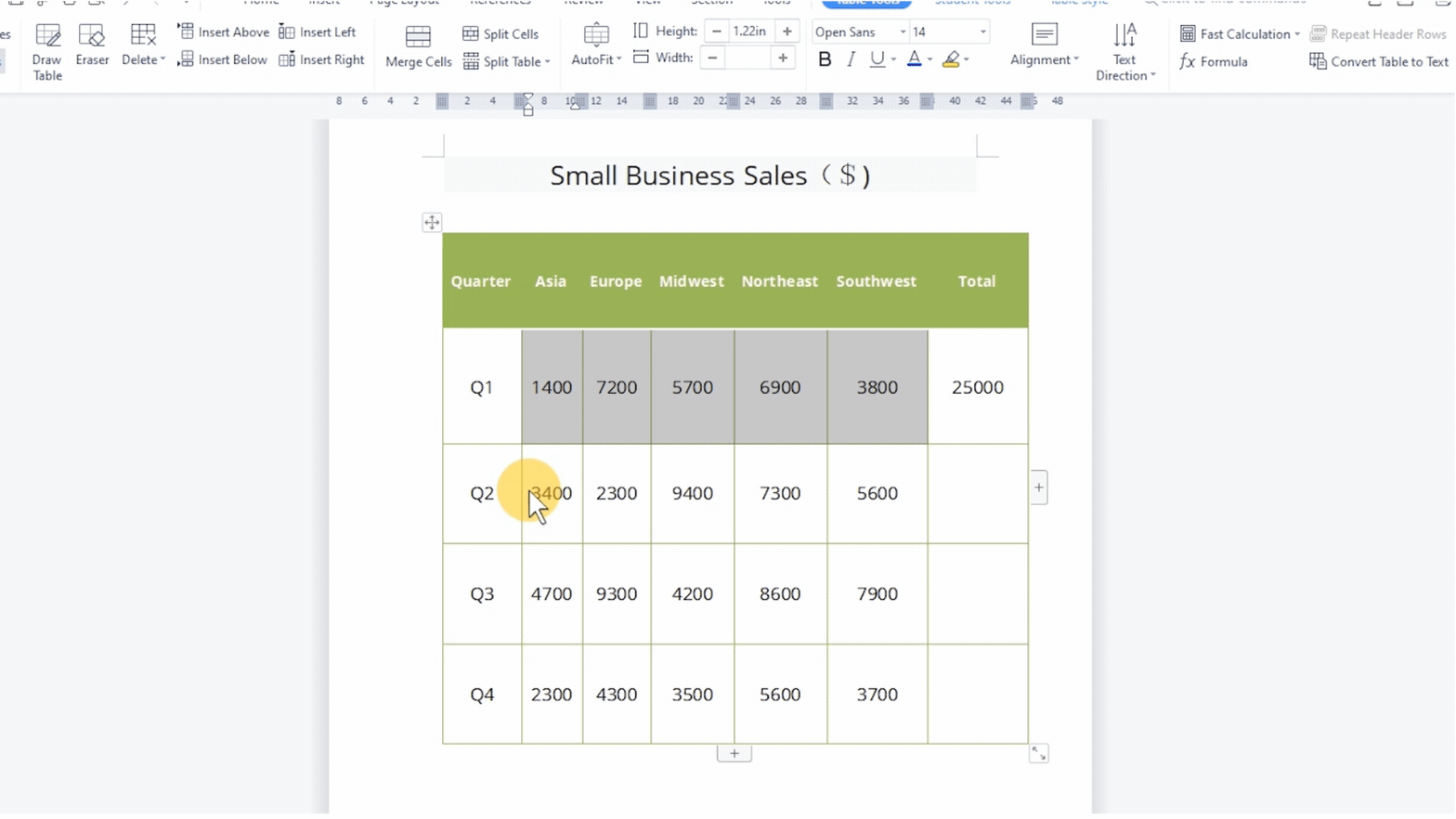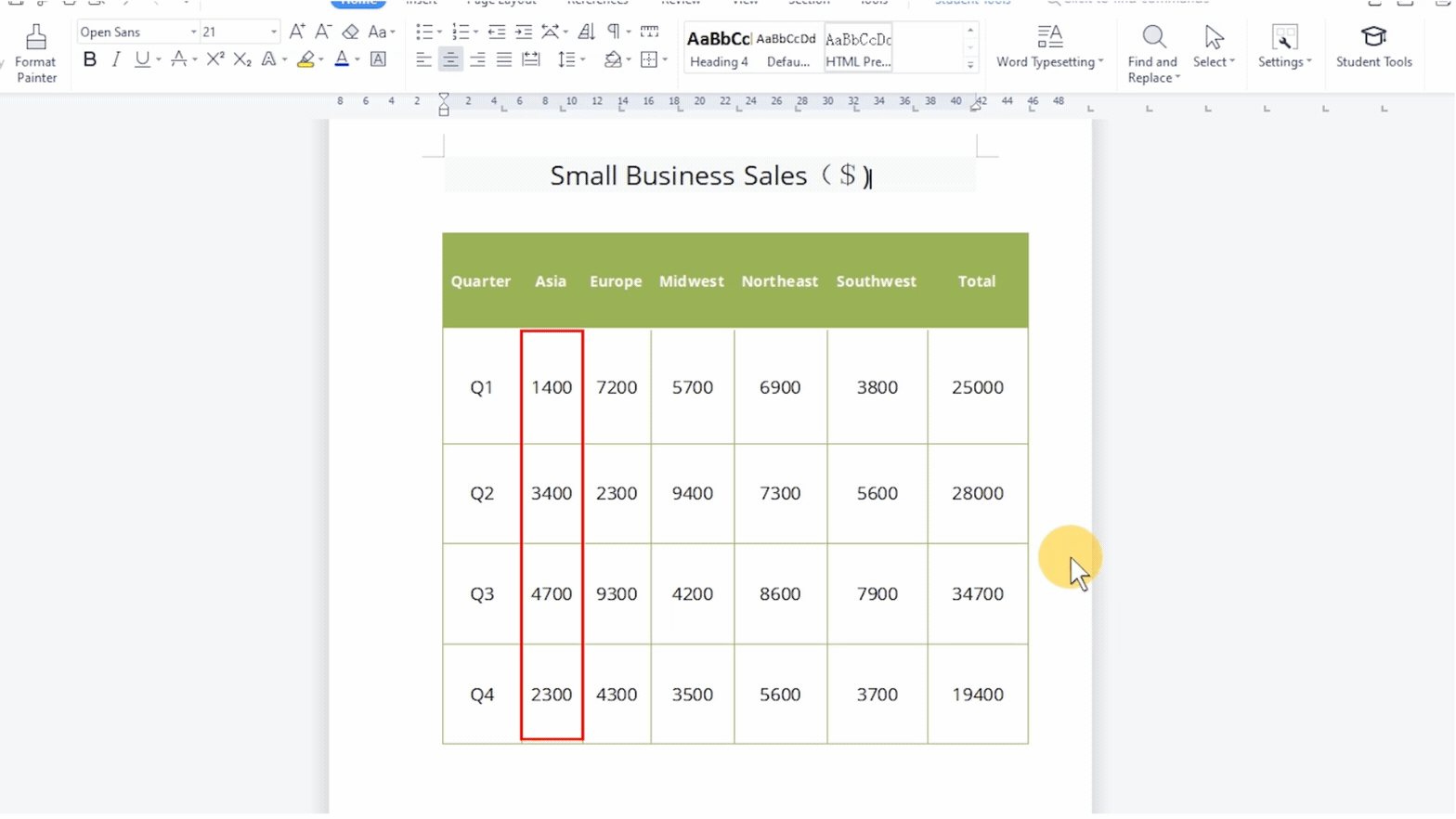# How to use the fast calculation function of table in WPS Writer

Uploaded time: February 28, 2022 Difficulty: Beginner# How to use the fast calculation function of table in WPS Writer

Do you know how to perform statistical operations on tabular data inserted in a document? It teaches you to perform quick calculations in a document in one minute.

For example, let's say we want to figure out the total sales of small businesses in Q1 for all regions.

First of all, insert a total column on the right of the table,select the data cell of row Q1, click the Table Tools tab, click the Fast Calculation drop-down button, and select Sum to calculate the Sum of the data in this row.Select the data cells in the remaining rows to quickly figure out the total sales for each quarter for all regions to the left.For example, find the total sales of small businesses in Asia. Using the same operation, you can calculate the sum of the data in this column.In addition, we can also use the Fast Calculation function to find the Average value of the table data, Max value, and Min value.Have you learned this simple and practical function?

WPS Office Spreadsheet is helpful for various fields of studies: mathematics, statistics, data science, engineering, finance, and such. Rich in functions paired with teaching procedures, WPS Office Spreadsheet definitely is a professional and reliable toolkit.